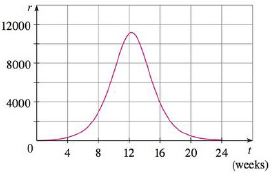Chapter 5, Problem 61RE### Single Variable Calculus: Early Tr...

8th Edition
James Stewart
ISBN: 9781305270343

#### Solutions

Chapter
Section### Single Variable Calculus: Early Tr...

8th Edition
James Stewart
ISBN: 9781305270343
Textbook Problem

# A population of honeybees increased at a rate of r(t) bees per week, where the graph of r is as shown. Use the Midpoint Rule with six subintervals to estimate the increase in the bee population during the first 24 weeks.To determine

To calculate: The increase of honeybee population during first 24 weeks.

Explanation

Given information:

Consider the number of subintervals as n=6.

The region lies between t=0 to t=24weeks.

Midpoint Rule:

abf(x)dxi=1nf(x¯i)Δx=Δx(f(x¯1)+.......+f(x¯n))

Calculate the width of subinterval using the formula.

Δx=ban (1)

Calculate the midpoint using the formula.

x¯i=12(xi1+xi) (2)

Here, width of the subintervals is Δx, the upper limit is b, the lower limit is a and the midpoint of (xi1,xi) is x¯i.

To find the width of the subintervals using Equation (1) as shown below.

Substitute 6 for n, 24 weeks for b and 0 for a in Equation (1).

Δt=2406=4

The expression to find the increase in bee population using midpoint rule as shown below.

024r(t)dtΔt(r(t¯1)+r(t¯2)+r(t¯3)+r(t¯4)+r(t¯5)+r(t¯6)) (3)

The end points of the six intervals are 0, 4 weeks, 8 weeks, 12 weeks, 16 weeks, 20 weeks and 24 weeks

### Still sussing out bartleby?

Check out a sample textbook solution.

See a sample solution

#### The Solution to Your Study Problems

Bartleby provides explanations to thousands of textbook problems written by our experts, many with advanced degrees!

Get Started

#### In Exercises 47-52, find and simplify f(a+h)f(a)h(h0) for each function. 52. f(x)=x

Applied Calculus for the Managerial, Life, and Social Sciences: A Brief Approach

#### In problems 23-58, perform the indicated operations and simplify. 26.

Mathematical Applications for the Management, Life, and Social Sciences

#### Given: ABDC DB bisects ADC m1=36 Find: mA

Elementary Geometry For College Students, 7e

#### Explain the distinction between science and pseudoscience.

Research Methods for the Behavioral Sciences (MindTap Course List)